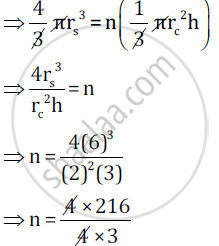Share

# A Certain Number of Metallic Cones, Each of Radius 2 Cm and Height 3 Cm Are Melted and Recast into a Solid Sphere of Radius 6 Cm. Find the Number of Cones. - ICSE Class 10 - Mathematics

ConceptArea and Volume of Solids - Sphere

#### Question

A certain number of metallic cones, each of radius 2 cm and height 3 cm are melted and recast into a solid sphere of radius 6 cm. Find the number of cones.

#### Solution

Let the number of cones be n.

Let the radius of the sphere be rs =6 cm

Radius of a cone be rc =2 cm

And the height of the cone be h =3 cm

A volume of sphere = n(Volume of a metallic cone)

=> 4/3 pir_s^3 = n(1/3 pir_c^2 h)n = 72

Hence, the number of cones is 72.

Is there an error in this question or solution?

#### APPEARS IN

2015-2016 (March) (with solutions)
Question 2.3 | 3.00 marks
Solution A Certain Number of Metallic Cones, Each of Radius 2 Cm and Height 3 Cm Are Melted and Recast into a Solid Sphere of Radius 6 Cm. Find the Number of Cones. Concept: Area and Volume of Solids - Sphere.
S